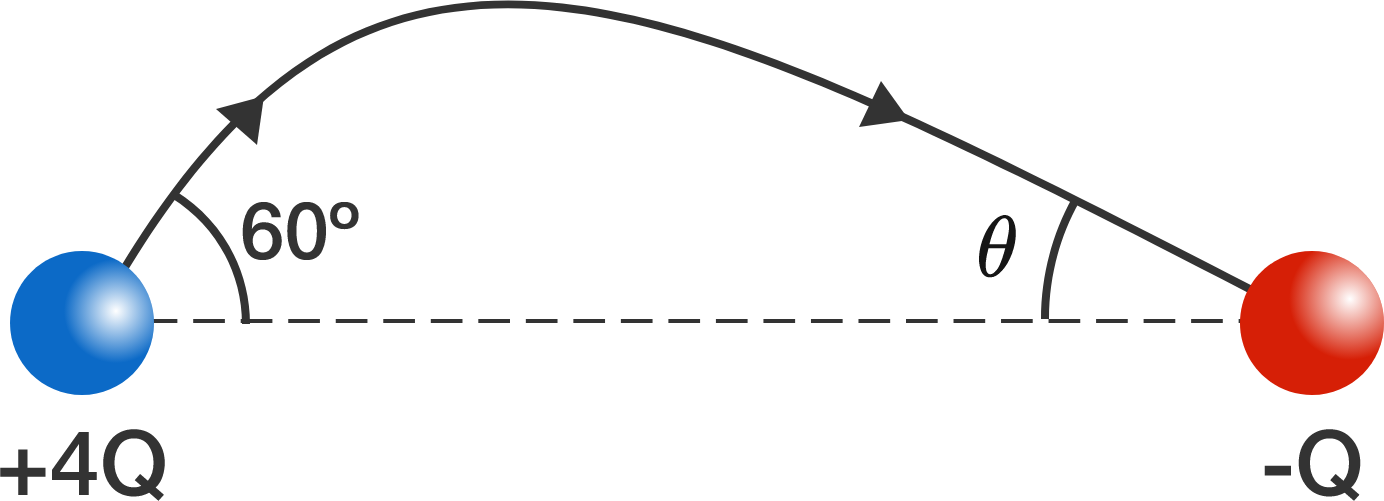# Electric AngleNote: the angles in the diagram are not drawn to scale.

An electric field line leaves a positive charge at an angle of $60^{\circ}$ and enters a negative charge at an angle of $\theta$. These angles are measured from the straight line joining the two charges.

The magnitude of the positive charge is 4 times the magnitude of the negative charge.

What is $\theta?$

×